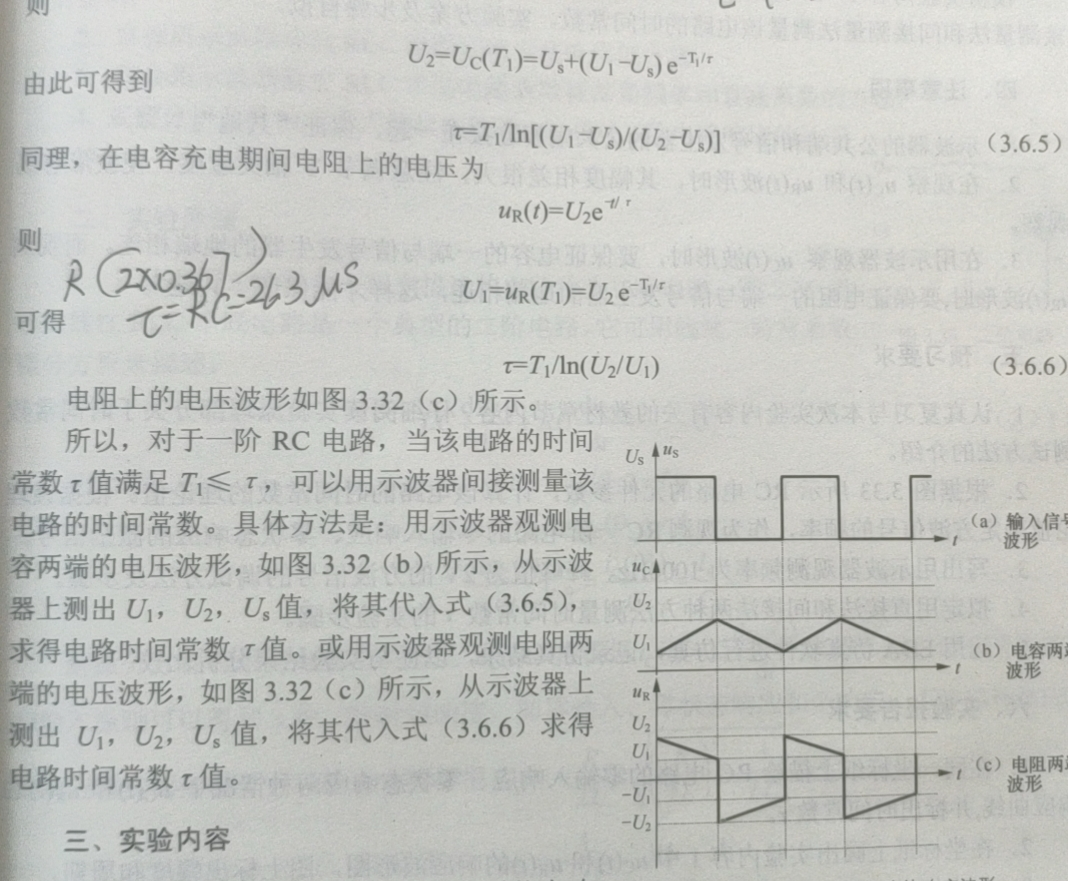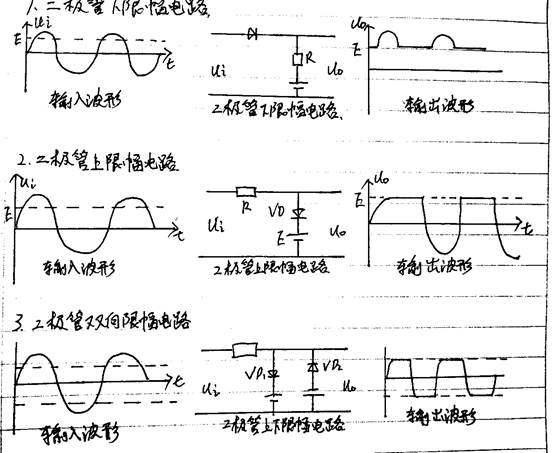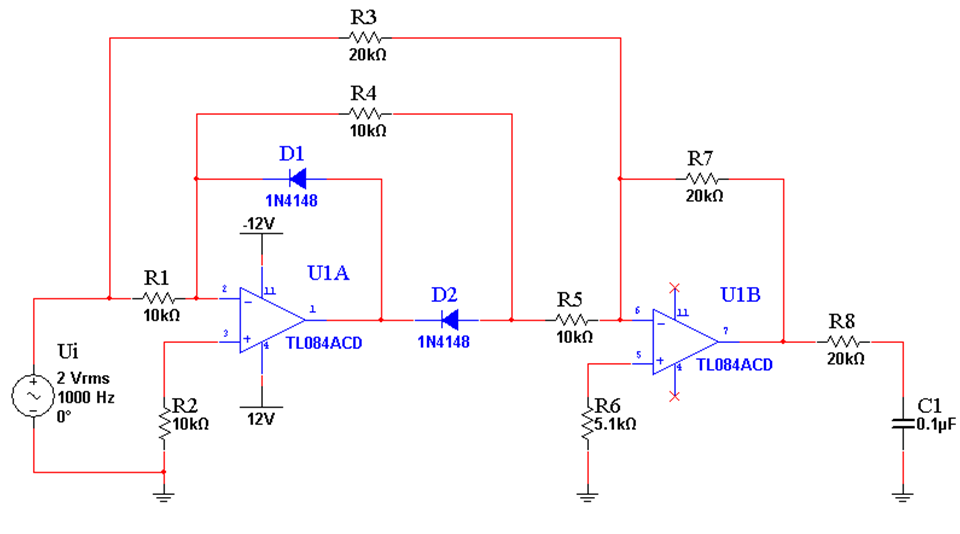# 电工电子实验复习

1. 理解各种仪表（万用表、示波器等）的测量值。如:幅值、峰峰值、有效值。
峰峰值（$V_{pp}$）：波形最高点和最低点的电压值
最大/小值（$V_{max}/V_{min}$）： 波形最高/最低点对$GND$（地）的电压值
幅值（$V_{amp}$）：波形顶部与底部的电压值
顶/底端值（$V_{top}/V_{base}$）： 波形顶/底部对$GND$（地）的电压值
均方根值（$V_{rms}$）：信号的有效
平均值（$Vaverage$）：信号的平均值
正/负占空比：正/负脉冲与周期的比值

2. 掌握各种仪表的使用方法。如:示波器触发源的选择（观测单踪和双踪信号稳定显示波形的方法步骤)、示波器的输入信号的耦合方式（DC耦合、AC耦合，两种耦合方式显示波形的区别);函数发生器如何产生一定频率和幅值的正弦波、三角波、方波;方波占空比的概念、调整函数发生器的占空比的方法;

• 示波器触发源的选择（观测单踪和双踪信号稳定显示波形的方法步骤)

• 单路波形
触发信源选择：Trigger->Menu->信源选择->CH1（CH2），然后调节level旋钮，使触发电平线落于所选通道波形上某个位置。（按下可归零）
• 双路波形
两路信号同频不同幅，选择幅度大的信号；两路信号同幅不同频，选择周期大的信号所接的通道。
• 示波器的输入信号的耦合方式（DC耦合、AC耦合，两种耦合方式显示波形的区别)

（按下 $CH1$ 按钮显示功能菜单选择耦合）
交流（AC）耦合：阻挡输入信号的直流成分
直流（DC）耦合：通过输入信号的交流和直流成分

• 函数发生器如何产生一定频率和幅值的正弦波、三角波、方波
正弦波：Sine
三角波：Ramp
方波：Square
有效值：$V_{rms}$
峰峰值：$V_{pp}$

• 方波占空比的概念、调整函数发生器的占空比的方法
正/负占空比：正/负脉冲与周期的比值

3. 掌握发光二极管、稳压管伏安特性的测量方法;会画稳压管的伏安特性曲线并知道稳压管的稳压区域。

• 发光二极管伏安特性的测量方法
• 稳压管伏安特性的测量方法
• 稳压管的伏安特性曲线
• 稳压管的稳压区域
4. 掌握戴维南等效参数（开路电压、等效电阻）的计算和测量方法。

• 开路电压$U_{oc}$：零示法
• 等效电阻$R_{eq}$：半电压法(从0开始调节电位器，电压表到$U_{oc}$一半时停止调节)
5. 掌握直接法测量一阶RC时间常数的方法;会计算时间常数的理论值;会根据电路时间常数选择输入信号的频率;会画电阻和电容两端的电压波形;并能在波形图上标注时间常数的坐标。

• 直接法测量一阶RC时间常数
取充电时的 $0.632U_s$ 到放电时的 $0.368U_s$ 在横轴上的投影长度即为时间常数 $\tau$
• 计算时间常数的理论值
$\tau = RC$
• 根据电路时间常数选择输入信号的频率 （???）
• 画电阻和电容两端的电压波形• 在波形图上标注时间常数的坐标 （???）
6. 掌握RLC串联谐振电路 $R, L, C, Q, fo, BW$ 的相互关系;掌握 $Q,fo,BW,URo,ULo, UCo$ 的理论值计算方法和测试方法;会画电流谐振曲线;能根据 $Q,fo,BW$等参数确定 $R,C,L$ 的值。

7. 掌握低通，高通，带通，带阻四种电路的频率特性，会画幅频，相频特性曲线;会计算低通，高通电路的截止频率的理论值;了解这些电路的实际应用。

• 幅频（Magnitude）曲线：${V_o\over V_i} - f$，
（高通、低通）半功率点：$(f_c,0.707)$，此时 $f_c$ 称为截止频率；
（带通）谐振点：$(f_0,1)$ （取最大值），谐振点频率
（带阻）固有点：$(f_0,0)$（取最小值），固有点频率
• 相频（Phase）曲线
• Multisim：
F为轴上最大值，I为最小值
8. 会根据要求输出要求设计限幅电路的电源参数;并会画出相应的输入输出波形。9. 掌握单级放大器的静态工作点，放大倍数，输入电阻，输出电阻等交流指标的理论值方法和测试方法;会根据要求设计计算放大器的电路参数（主要是电阻）了解各电路参数与静态工作点、交流指标之间的关系﹔改变静态工作点会引起哪两种失真，调节哪些参数可以消除失真。会正确记录输入、输出波形以及失真波形。

10. 会计算差分放大器的静态工作点，差模放大倍数和共模放大倍数的理论值;掌握差模放大倍数和共模放大倍数的测量方法，正确记录差模和共模输入、输出波形（交流耦合情况下），并标注完整。

• 操作题：差动放大器（仪器、运放、电分实验）
• $A_{ud}={U_{od}\over U_{id}}= {U_{c1}+U_{c2}(有效值相加)\over U_{i1}(有效值) } \approx 4$
$A_{uc} ={U_{oc}\over U_{id}}= {U_{c1}-U_{c2}(有效值相减)\over U_{i1}=(有效值U_{i1}=U_{i2}) } \approx 0$
$K_{CMR}=\left|{A_{ud}\over A_{uc}}\right|\approx \infty$
11. 会正确使用TL084运算放大器，会用实验的方法检测运算放大器的好坏;能运用运算放大器设计反相加法器、反相积分器，会设计计算各个电路参数;会用示波器测量输入、输出波形的峰峰值、平均值和周期、频率等参数;能正确记录实验结果，画出输入、输出波形,并标注完整。

• TL084集成运放
4接正，11接负，电源由近及远为：$+ , -,\infty$ ，本实验电源采用 $\pm 9V$

• 操作题：反相加法器（仪器、模电实验、电分实验）
$U_o = -({R_3\over R_1}\times U_{i1}+{R_3\over R_2}\times U_{i2})$
$R_4=R_1//R_2//R_3$

• 操作题：反相积分器（仪器、模电实验、电分实验）
$U_o = -{1\over RC}U_st$
$R_f=10R, R_p = R//R_f$

$C\ge \tau/R$，其中 $t = T/2,\tau= RC \ge {U_s\over U_{omax}}t$

12. 掌握方波-三角波发生器的工作原理及各元件的作用;会设计计算各个电路元件参数。用示波器测量输出波形的上下峰值和频率;能正确记录输出波形。

• 操作题：波形变换电路（强化班加用）（仪器、模电实验、电分实验）

一般来说，$I_{Dzmax}=10mA$
方波输出幅度决定二极管的稳压值 $V_z = 方波输出幅度V$
误差范围 $\pm x\%\Rightarrow U_{o1min}= (方波输出幅度 - 方波输出幅度\times x\%)V$
$R_3 = (U_s-U_{o1min})/I_{Dzmax}=…$
$U_{omax} =方波输出幅度V$
$U_{omax}={R_1\over R_f}(U_z+U_D)$，得到$R_1,R_f$比例关系
$R_1$不能太小，可以取 $5.1k\Omega$
$R_p=R_1//R_f$
$R_4 = {R_f\over 4CR_1f}$ ，$f$ 为振荡频率，$C$ 可取 $0.01uF\sim 1uF$ ，然后确定$R_4$
通常取 $R_5=R_4$

13. 掌握精密整流电路和电压比较器的工作原理，会根据输出波形设计精密整流电路各个参数，会正确记录精密整流电路各部分的输出波形。

• 操作题：精密整流电路（仪器、模电实验、电分实验）（运放电源下正上负）
当 $U_i>0$时，$R_7/R_3=1，R_7/R_5=-2$ 即： $U_{o2}=-(U_i+2(-U_i)) = U_i$
当$U_i<0$时，只有 $U_i$ 输入，故 $U_{o2}=-U_i$